# MCAT Physical : Enthalpy

## Example Questions

### Example Question #1 : Enthalpy

The formation of nitrous oxide is a 2-step process.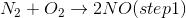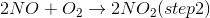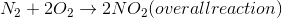The overall enthalpy of the reaction is +68kJ.

If the enthalpy change of step 1 is 180kJ, what is the enthalpy change of step 2?

248kJ

112kJ

–112kJ

–112kJ

Explanation:

Hess's law states that the enthalpies of the steps in an overall reaction can be added in order to find the overall enthalpy. Since the overall enthalpy of the reaction is +68kJ, we can use the following equation to find step 2's enthalpy.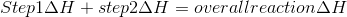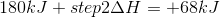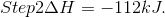### Example Question #2 : Enthalpy

Consider the following processes:

1)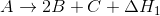2)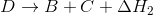3)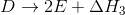Which for the following expresses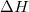for the process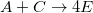?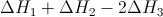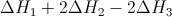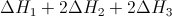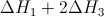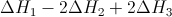Explanation:

1)2)3)We need to add the given processes in such a way that leaves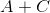on the left side, and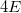on the right.

Since there are no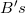in the final equation, begin by combining processes 1 and 2 to eliminate.

First, reverse process 2 and multiply by two:

2a)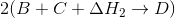2a)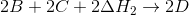2a)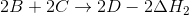Add this result to process 1.

1)2a)1+2a)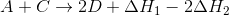Now we need to combine with process 3 to eliminate the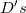. Start by multiplying process 3 by two.

3a)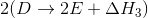3a)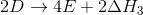Add this to the combined result from processes 1 and 2.

1+2a)3a)1+2a+3a)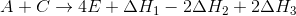This gives our final combination. The elemental reaction is, and the enthalpy of the process is.